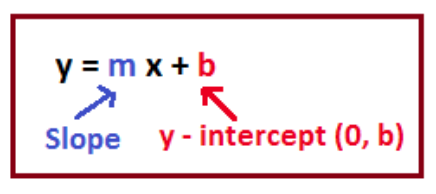# How do you find the slope and intercept of 6x - 14 = y?

Aug 3, 2018

Slope: $6$

x-intercept: $\left(\frac{7}{3} , 0\right)$

y-intercept: $\left(0 , - 14\right)$

#### Explanation:

$y = 6 x - 14$

This equation is in slope-intercept form:Based on the image, we know that the slope is the value multiplied by $x$, so the slope is $6$.

We know the $y$-intercept is $b$, or $- 14$, so the $y$-intercept is at $\left(0 , - 14\right)$.

To find the $x$-intercept, plug in $0$ for $y$ and solve for $x$:
$0 = 6 x - 14$

$14 = 6 x$

$\frac{14}{6} = x$

$\frac{7}{3} = x$

$x = \frac{7}{3}$

The $x$-intercept is at $\left(\frac{7}{3} , 0\right)$.

Hope this helps!

Aug 3, 2018

Slope $6$, $x$-int $\frac{7}{3}$, $y$-int $- 14$

#### Explanation:

We have the following:

$y = 6 x - 14$

The good thing is that this equation is in slope-intercept form

$y = m x + b$, with slope $m$ and a $y$-intercept of $b$.

From pattern matching, we see that our slope is $6$ and our $y$-intercept is $- 14$. We find our $x$-int. by setting $y$ to zero.

We get

$6 x - 14 = 0 \implies 6 x = 14 \implies x = \frac{7}{3}$

Therefore, our slope is $6$, our $y$-intercept is $- 14$ and our $x$-intercept is $\frac{7}{3}$.

Hope this helps!